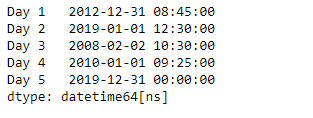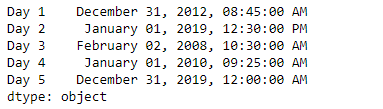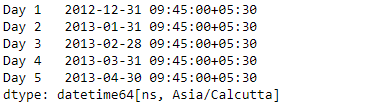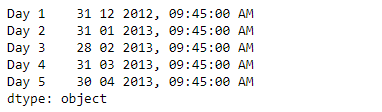# Python | Pandas Series.dt.strftime

`Series.dt` can be used to access the values of the series as datetimelike and return several properties. Pandas` Series.dt.strftime()` function is used to convert to Index using specified date_format. The function return an Index of formatted strings specified by date_format, which supports the same string format as the python standard library.

Syntax: Series.dt.strftime(*args, **kwargs)

Parameter :
date_format : Date format string (e.g. “%Y-%m-%d”)

Returns : Index of formatted strings

Example #1: Use `Series.dt.strftime()` function to convert the dates in the given series object to the specified date format.

 `# importing pandas as pd ` `import` `pandas as pd ` ` `  `# Creating the Series ` `sr ``=` `pd.Series([``'2012-12-31 08:45'``, ``'2019-1-1 12:30'``, ``'2008-02-2 10:30'``, ` `               ``'2010-1-1 09:25'``, ``'2019-12-31 00:00'``]) ` ` `  `# Creating the index ` `idx ``=` `[``'Day 1'``, ``'Day 2'``, ``'Day 3'``, ``'Day 4'``, ``'Day 5'``] ` ` `  `# set the index ` `sr.index ``=` `idx ` ` `  `# Convert the underlying data to datetime  ` `sr ``=` `pd.to_datetime(sr) ` ` `  `# Print the series ` `print``(sr) `

Output :Now we will use `Series.dt.strftime()` function to convert the dates in the given series object to the specified format.

 `# convert to the given date format ` `result ``=` `sr.dt.strftime(``'% B % d, % Y, % r'``) ` ` `  `# print the result ` `print``(result) `

Output :As we can see in the output, the `Series.dt.strftime()` function has successfully converted the dates in the given series object to the specified format.

Example #2 : Use `Series.dt.strftime()` function to convert the dates in the given series object to the specified date format.

 `# importing pandas as pd ` `import` `pandas as pd ` ` `  `# Creating the Series ` `sr ``=` `pd.Series(pd.date_range(``'2012-12-31 09:45'``, periods ``=` `5``, freq ``=` `'M'``, ` `                            ``tz ``=` `'Asia / Calcutta'``)) ` ` `  `# Creating the index ` `idx ``=` `[``'Day 1'``, ``'Day 2'``, ``'Day 3'``, ``'Day 4'``, ``'Day 5'``] ` ` `  `# set the index ` `sr.index ``=` `idx ` ` `  `# Print the series ` `print``(sr) `

Output :Now we will use `Series.dt.strftime()` function to convert the dates in the given series object to the specified format.

 `# convert to the given date format ` `result ``=` `sr.dt.strftime(``'% d % m % Y, % r'``) ` ` `  `# print the result ` `print``(result) `

Output :As we can see in the output, the `Series.dt.strftime()` function has successfully converted the dates in the given series object to the specified format.

My Personal Notes arrow_drop_upCheck out this Author's contributed articles.

If you like GeeksforGeeks and would like to contribute, you can also write an article using contribute.geeksforgeeks.org or mail your article to contribute@geeksforgeeks.org. See your article appearing on the GeeksforGeeks main page and help other Geeks.

Please Improve this article if you find anything incorrect by clicking on the "Improve Article" button below.

Article Tags :

Be the First to upvote.

Please write to us at contribute@geeksforgeeks.org to report any issue with the above content.